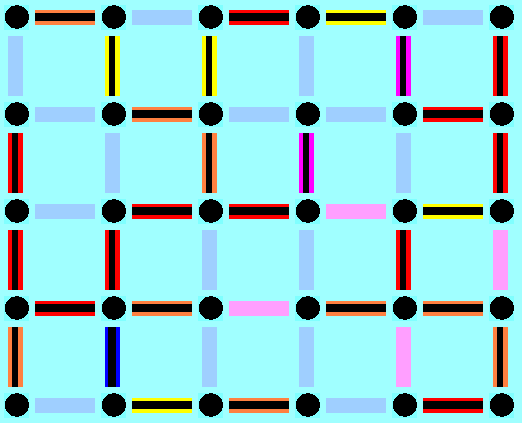CMIS 160
Discrete Mathematics

Erich Prisner
UMUC SG
Spring 2002

# Project #3

This project is due on Thursday, March 22 (firm deadline).

## 1. Another Brick in the Wall

Your task is to determine the number of different possible walls. We consider only walls that have height 2 units, built only by bricks of dimension 2 to 1. Vertical bricks come in two colors (black, red, orange), and horizontal bricks in two colors (blue and orange). Each color has its own price: 3 euro for black, 2 euro for blue, 2 euro for red, and 1 euro for orange. With the applet you can build walls of a certain length and total cost, by clicking on the six symbols on the bottom. Click on "away" for removing the last brick, and on the other 5 symbols for adding the corresponding brick (if possible) at the end of the wall.

Unfortunately your browser does not support Java applets.a) First we don't care about the colors. Then there is only one wall of length 1, two of length 2, and three of length 3. Here are 3 walls of length 7. The question is: How many different walls of length 6 are there (where walls of the same shape but different colors are considered to be the same here)? List all these shapes.b) How many different uncolored walls of length n are there? Find a recurrence equation between the numbers. Solve the recurrence equation.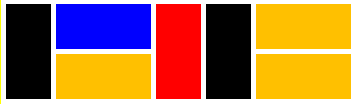c) Now we recognize color. The two length 7 walls to the right are therefore now considered different, although we didn't distinguish between them in parts (a) and (b). How many different colored walls of length 7 are now possible? This time it is not necessary to list all these walls.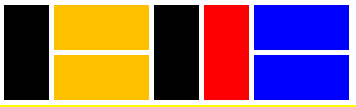d) What is the general formula for the number of walls of length n now? Again use recurrence equations.

e) Finally not the length but the total cost (defined as the sum of the cost of all bricks) of the walls is the issue. To the right you see three different walls of total cost 10 each. How many different walls of total cost 5 are there?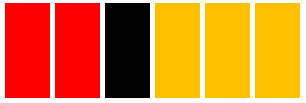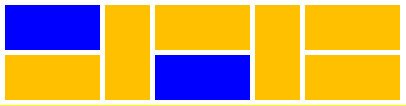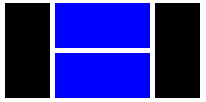f) And of course, the final question is about the number cn of walls of fixed cost n Find a recurrence equation. Find the corresponding characteristic equation---it is now a polynomial equation of degree 4. Find the zeros of the polynomial using your Math 107 knowledge on polynomials and the
Then find a formula for the number cn of walls of fixed cost n.

Go to Project # 4.
Go back to Project # 2.

Projects differ in certain ways from homework. They questions there are more complex, more difficult, have several steps, and sometimes you have to use notions and tools from several chapters to solve them. But in most cases, the theorems and tools learned do not suffice to solve all parts of the project. Rather you have to discover certain facts by yourself. Learning how to make "discoveries" in the field is an important part of the class---in real life you don´t have always a textbook telling you exactly what to do. Of course you are encouraged to use any other material---books, webpages, and so on.